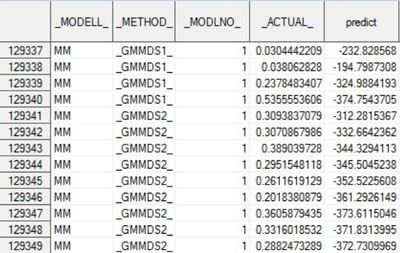## Big difference between actual and predicted values in GMM Estimation

Hi Expert Fellas;

I have a panel of some firms (GVKEY) in a period of time (FYEAR) with some variables related to them. here is a link to a sample of my data (real database has more than 60000 rows):

https://www.dropbox.com/s/hpphgpluknk5414/Data%20Sample.csv?dl=0

I tried to run a GMM Estimation using the following code:

``````proc panel data=Lev_lag outest=outst noprint;
output out=outx PREDICTED=predict;
inst depvar(LEVEL);
MM: model Lev = Lev_1 MTBV Size Tangibility Profitability Cashes  Tax  TB3Ms
/ gmm nolevels twostep maxband=3 noint fixtwo  ;
id gvkey fyear;
run; ``````

"outx" is an output that shows both actual and predicted values of my model. now, the problems are:

1. as it is depicted in the following picture, there is a huge difference between actual and predicted values of my dependent variable. this is, while all the variables have significant coefficients in the model.2. I wanted to have a GMM model with Fixed effect for both Year and Firm. So, I added "fixtwo" to my model options. However, the output file shows me another result only for Fixtwo model which is seperated from the GMM results!as you can see, the results for fixed effect model are logical, and actual model is pretty similar to the predicted model (good fit). However, It's not GMM anymore!

Now, would you please guide me through this problem? I'm not sure what step of my work is wrong which gives me this model.

thanks everybody.

2 REPLIES 2

## Re: Big difference between actual and predicted values in GMM Estimation

Hello,

I run your model using the sample data you provided. Here are few suggestions that you might want to try.

1. FIXTWO option has no impact on estimating the GMM model. Basically it just estimates FIXTWO model in addition to GMM one.

2. DEPVAR(LEVEL) and NOLEVELS options are conflicting. DEPVAR(LEVAL) says to use levels as instruments while NOLEVELS is saying to ignore it. In the end NOLEVELS is ignored and it estimates the model with levels as instruments only.

3. Is this the correct model you want to estimate with levels as instruments only? You might want to use differenced equations too. See the DEPVAR option in SAS/ETS documentation.

4. The first step is estimable (see this by removing TWOSTEP option) but using your sample data there is a problem with residuals. Is this model parametrized correctly?

5. Using the sample data the model fit is pretty bad (t-values close to zero). Likely that this parametrization is causing problems in 4.

Jan

## Re: Big difference between actual and predicted values in GMM Estimation

Thanks for your suggestions dear Jan.

I am working on the GMM model and my data sample, for over a month. I'm almost sure about parameterization of the model since it's a well-known model which I just try to replicate. there is only one thing in GMM model which was interesting for me. this model is highly sensitive to outliers. after I winsorized the data, the model fit surprisingly became much better. This is, while winsorizing didn't have a significant effect on FIXTWO results.

referring to #4, I got this error too and it seems that there is a multicollinearity in my model. However, I couldn't find it!! I could solve this problem by increasing the MAXBAND in GMM or omitting some specific variables from the model.

Overall, I found GMM a very sensitive model which its output can change easily and drastically by model's options like type of instruments, number of lags or maxband.